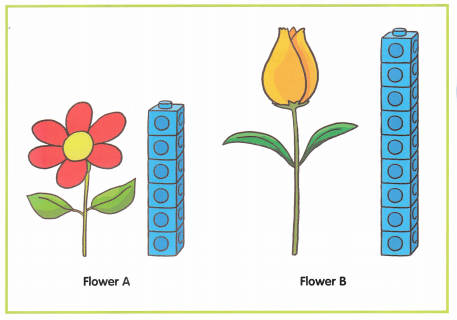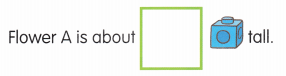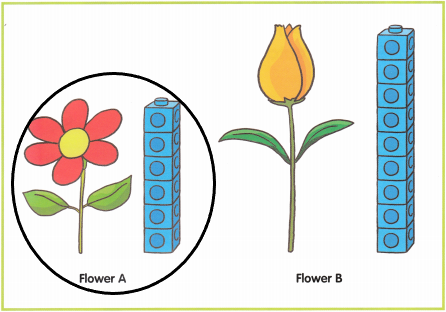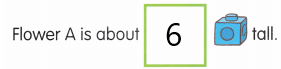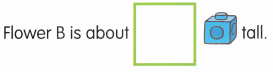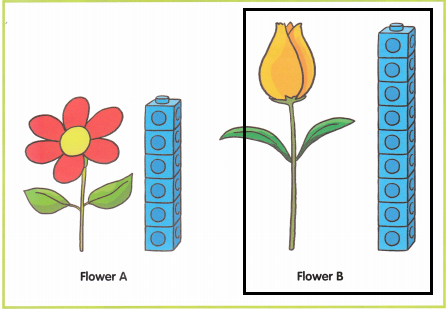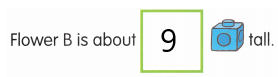# Math in Focus Kindergarten Chapter 15 Answer Key Length and Height

Go through the Math in Focus Grade K Workbook Answer Key Chapter 15 Length and Height to finish your assignments.

## Math in Focus Kindergarten Chapter 15 Answer Key Length and Height

Lesson 1 Comparing Lengths

Draw a long tail.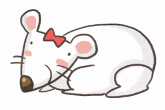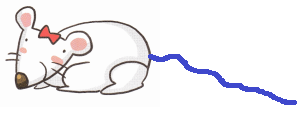The question asked was to draw a long tail.

Draw a short tail.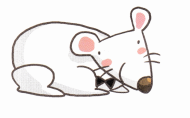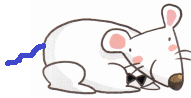The question asked was to draw a short tail.

Draw a longer object.

Question 1.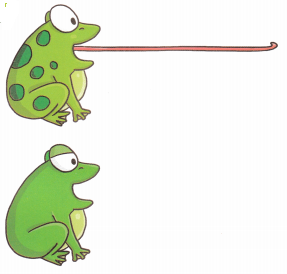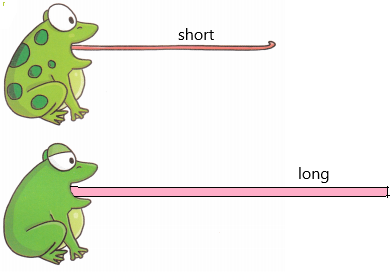Question 2.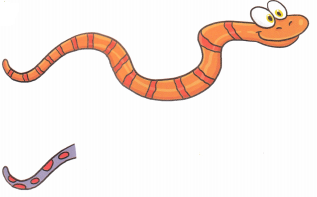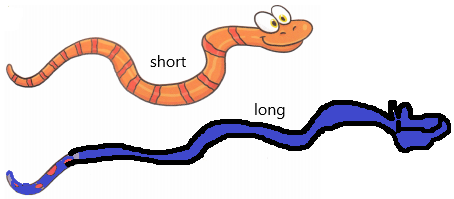Draw a shorter object.

Question 1.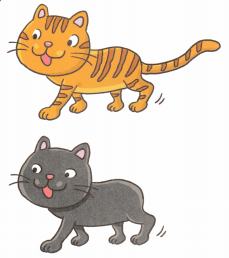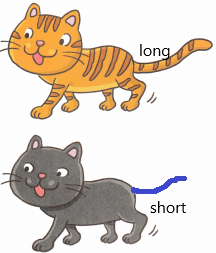Question 2.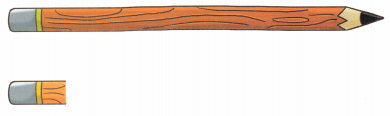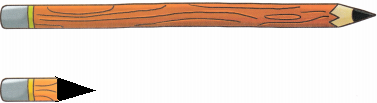Make an X on the kite with the longest tail. Circle the kite with the shortest tail.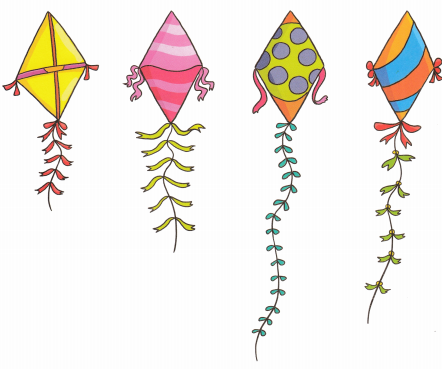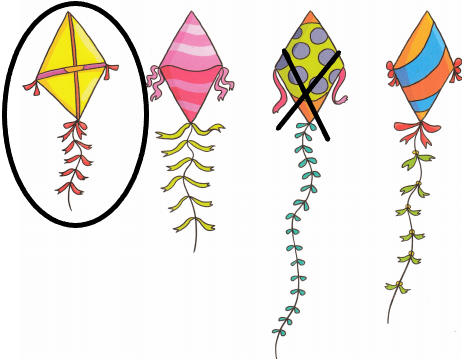Lesson 2 Comparing Lengths Using Nonstandard Units

Measure, count and write.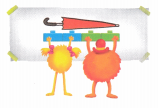Question 1.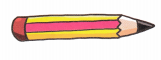The pencil is about ___________ cubes long.
The long and short scales are two of several naming systems for integer powers of ten which use some of the same terms for different magnitudes.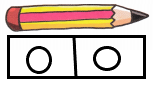The pencil is 2 cubes long.

Question 2.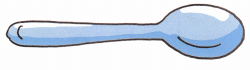The spoon is about ___________ cubes long.
The long and short scales are two of several naming systems for integer powers of ten which use some of the same terms for different magnitudes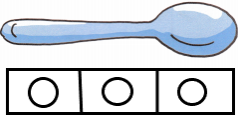The spoon is 3 cubes long.

Question 3.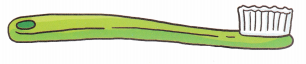The toothbrush is about ____________ cubes long.
The long and short scales are two of several naming systems for integer powers of ten which use some of the same terms for different magnitudes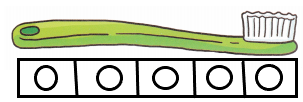The tooth brush is about 5 cubes long.

Question 4.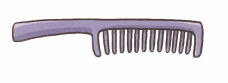The comb is about ____________ cubes long.
The long and short scales are two of several naming systems for integer powers of ten which use some of the same terms for different magnitudes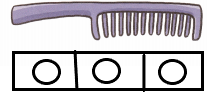The comb is about 3 cubes long.

Question 5.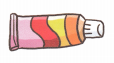The tube is about ____________ cubes long.
The long and short scales are two of several naming systems for integer powers of ten which use some of the same terms for different magnitudes.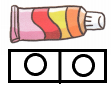The tube is about 2 cubes long.

Question 6.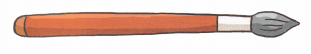The paintbrush is about ____________ cubes long.
The long and short scales are two of several naming systems for integer powers of ten which use some of the same terms for different magnitudes.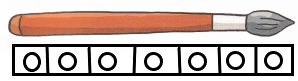The paint brush is about 7 cubes long.

Count and write.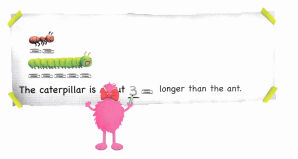Question 1.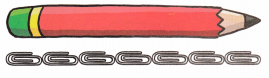The pencil is about __________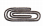long.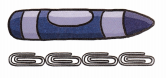The crayon is about ____________long.
The pencil is about ____________longer than the crayon.
The pencil is about 7 cubes long.
The crayon is about 4 cubes long.
The pencil is about ‘X’ longer than the crayon.
X=7-4
X=3
The pencil is about 3 cubes longer than a crayon.

Question 2.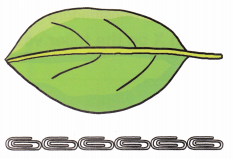The leaf is about ___________long.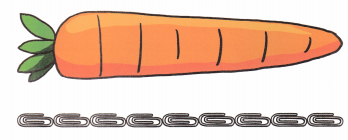The carrot is about __________long.
The leaf is about ____________shorter than the carrot.
The leaf is about 6long
The carrot is about 9long.
The leaf is about ‘X’shorter than the carrot.
X=9-6
X=3
The leaf is about 3shorter than the carrot.

Lesson 3 Comparing Heights Using Nonstandard Units

Count and write. Make an X on the taller vase.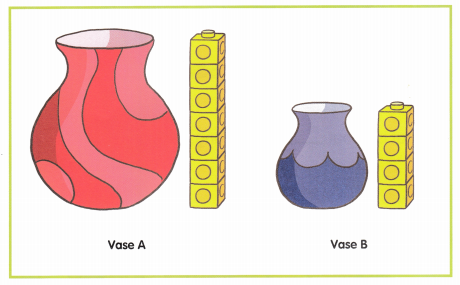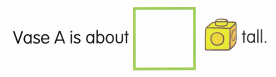I crossed vase A because it is a taller one.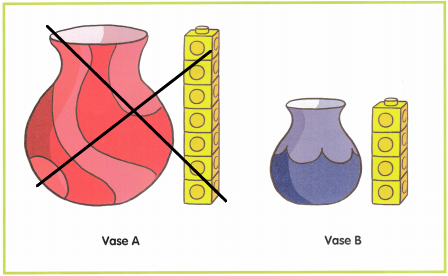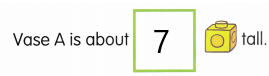Count the circles present in vase A.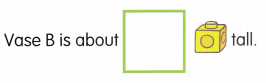Count the circles in vase B.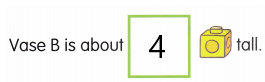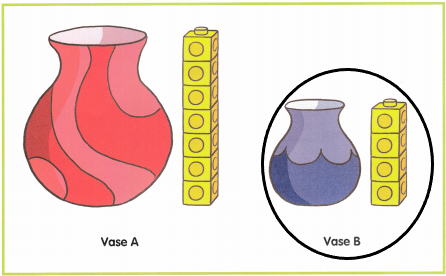Count and write. Circle the shorter flower.